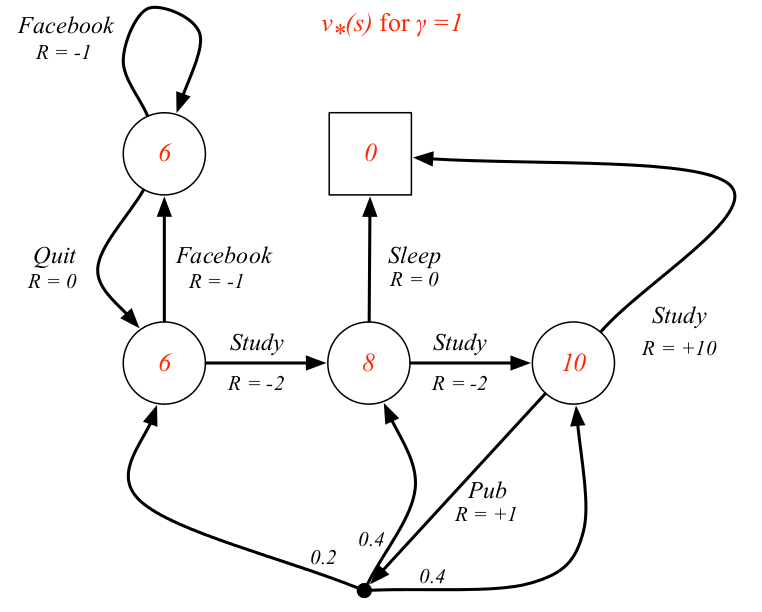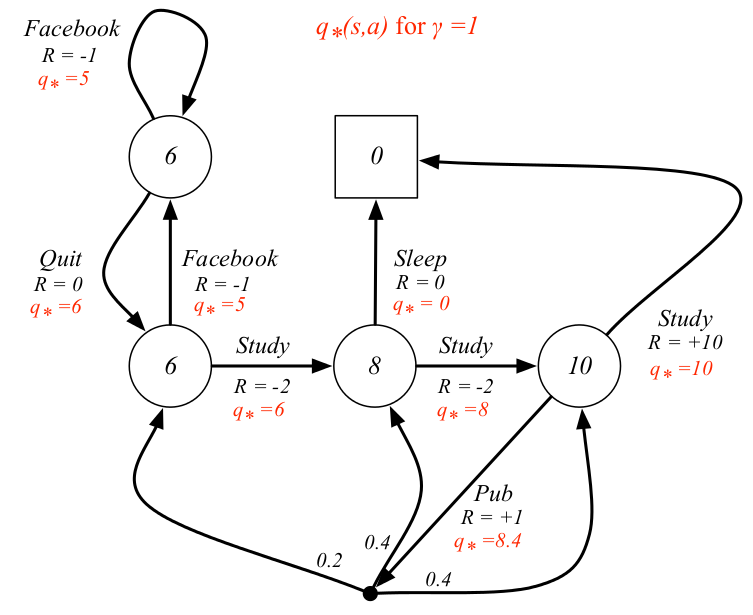【转载请注明出处】chenrudan.github.io#### 1.基础概念

reward函数ER: 这个函数是immediate reward的期望，即在时刻t的时候，agent执行某个action后下一个时刻立即能得到的reward $R_{t+1}$的期望，它由当前的状态决定。状态s下immediate reward期望为$ER_s = E[R_{t+1}|S_t = s]$，状态s下执行动作a后immediate reward期望为$ER_s^a = E[R_{t+1}|S_t=s, A_t=a]$

Return $G_t$与discount $\gamma$: $G_t$是t时刻之后未来执行一组action能够获得的reward，即t+1、t+2、t+3…未来所有时刻reward之和，是未来时刻reward在当前时刻的体现，但是越往后的时刻它能反馈回来的reward需要乘以一个discount系数，系数$\gamma \in [0,1]$会产生一个打折的效果，这是因为并没有一个完美的模型能拟合出未来会发生什么，未来具有不确定性，同时这样计算会方便，避免了产生状态的无限循环，在某些情况下，即时产生的reward即$R_{t+1}$会比未来时刻更值得关注，符合人的直觉。因此$G_t = R_{t+1}+\gamma R_{t+2}+…=\sum_{k=0}^{\infty}\gamma^kR_{t+k+1}$

#### 2. MDP与实例分析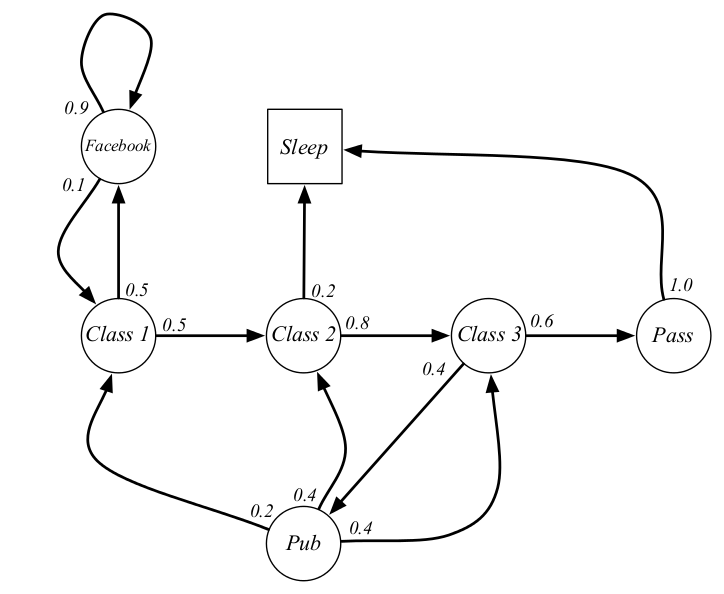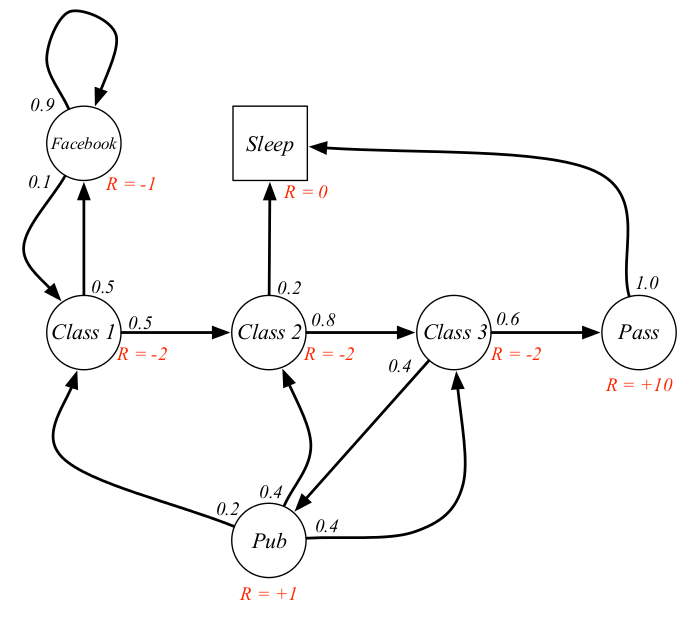$$\begin{split} v(s) = &E[G_t|S_t=s] \ = &E[R_{t+1} + \gamma (R_{t+2}+\gamma R_{t+3}+…) | S_t=s] \ = &E[R_{t+1} + \gamma G_{t+1} | S_t=s] \ = &ER_s + \gamma \sum_{s’\in S}P_{ss’}v(s’) \end{split} ::::: (1)$$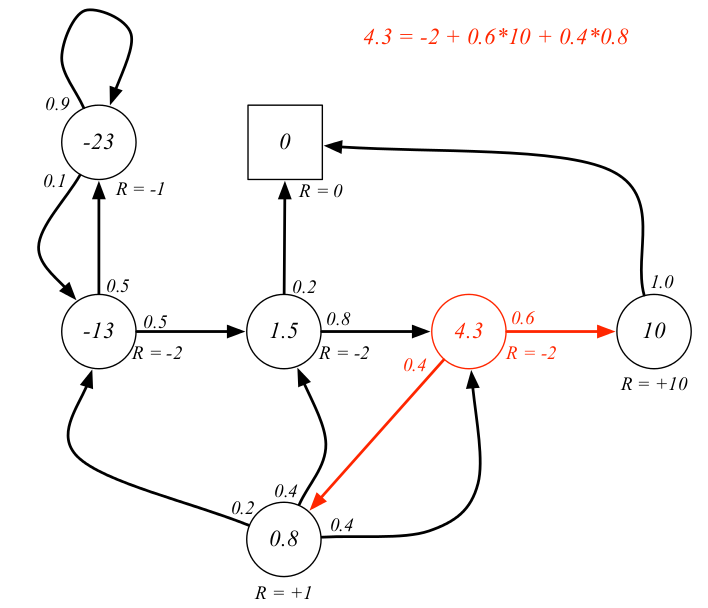$$v_{\pi}(s) = \sum_{a\in A}\pi(a|s)(R_s^a + \gamma \sum_{s’\in S}P_{ss’}^av_{\pi}(s’)) ::::: (2)$$

$$q_{\pi}(s,a) = R_s^a + \gamma \sum_{s’\in S}P_{ss’}^a\sum_{a’\in A}\pi(a’|s’)q_{\pi}(s’,a’) ::::: (3)$$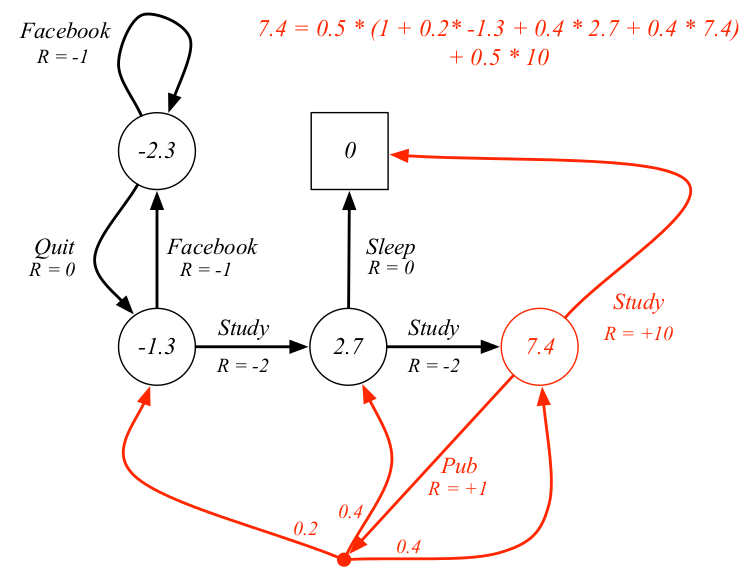$$v_*(s) = \underset{a}{max}: q_*(s,a) ::::: (4)$$

$$q_*(s,a) = R_s^a + \gamma \sum_{s’\in S}P_{ss’}^a \underset{a’}{max}: q_*(s’, a’) ::::: (5)$$